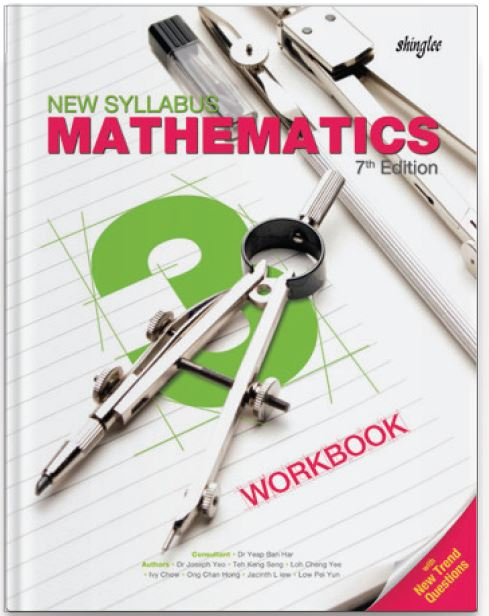Our prices are denominated in USD.

We ship internationally!# New Syllabus Mathematics Workbook 3 (7th Ed)

\$11.25

New Syllabus Mathematics is the most widely-adopted series of secondary mathematics textbooks in Singapore. Following the three-part lesson approach, pupils begin with an anchor task, followed by a guided practice, before concluding with an independent practice. New concepts are introduced and built upon in the textbooks. Well-structured exercises developed in
accordance to the lesson objectives are found in the workbooks.

This is a 4-year course in preparation for Cambridge O-level.

This is a 4-year course.

For Secondary 3 / Grade 9 / Year 9

Completing the Square, Formula, Graphical Method ad Fractional Equations

2. Linear Inequalities
Including Simultaneous Linear Inequalities
3. Indices and Standard Form
Including Compound Interest

4. Coordinate Geometry
Gradient, Length and Equation of Straight Line

5. Graphs of Functions and Graphical Solution
Cubic, Reciprocal and Exponential Functions

6. Further Trigonometry
Sine and Cosine of Obtuse Angles and Area of Triangle

7. Applications of Trigonometry
Angles of Elevations and Depression, Bearings and Three-Dimensional Problems

8. Arc Length, Area of Sector and Radian Measure

9. Congruence and Similarity Tests
Including Applications

10. Area and Volume of Similar Figures and Solids

11. Geometrical Properties of Circles
Symmetric and Angle Properties of Circles